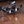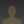0%
0%

# Contactless Loop Controller

Box to control the loop for metal stamping process.

Similar projects worth following
383 views
Contactless Loop Controller

I had made a box to control the metal loop several years ago, and wanted to revisit the project to improve the design and make it more robust.

The project originally started because the use of spring loaded sensing wires was not the best solution, but was what was available.  After pricing some of the ultrasonic sensors available for industry (currently between ~\$330 -\$1400) I thought that I could do better, and if not then one could always be purchased.  I built the original loop controller for less than \$100.  All of the electronics came from radioshack and a local electrical supply store, this way repairs could be made that day instead of waiting for a part. The frame was built from scrap metal.

• ### Note:

I tested objects with different colors, but not necessarily different reflectance. I will check the sensor shortly on highly reflective material, and make sure that the curve still fits.

• ### Caution

The sensor is very sensitive, and not having the object being detected parallel to the sensor can create a measurement +/- a couple of inches from the correct value.

• ### The code

```#Python Code to capture data points:

import serial
import mDb

class Looper():

def __init__(self):
host = "localhost"
dbName = "loop_controller"

self.checkTable("ir_cal_data2", [
{"name": "id", "type": "INT NOT NULL AUTO_INCREMENT PRIMARY KEY"},
{"name": "distance", "type": "TEXT"},
{"name": "pin_measurement", "type": "TEXT"}])

self.ser = serial.Serial(port='COM17', baudrate=9600)
self.ser.flushInput()
self.ser.flushOutput()

distance = 8
while distance < 61:
raw_input("Press Enter when ready for next reading.  Currently at " + str(distance) + ".")
self.log_data(distance)
distance += 1

def checkTable(self, table, data):
try:
self.db.beginTransaction()
self.db.checkTable(table, data)
self.db.commitTransaction()
except Exception as e:
print("WARNING")
print(e)

def log_data(self, distance):
counter = 0
data_raw = None
minVal = 0
maxVal = 0
avgVal = 0
firstVal = True
while counter < 250:
try:
data_raw = int(data_raw)
data = {"distance": str(distance), "pin_measurement": str(data_raw)}

try:
self.db.beginTransaction()
self.db.insert("ir_cal_data2", data)
self.db.commitTransaction()
except Exception as e:
print("WARNING")
print(e)

if firstVal:
firstVal = False
minVal = data_raw
maxVal = data_raw
avgVal += data_raw
else:
if data_raw < minVal:
minVal = data_raw
if data_raw > maxVal:
maxVal = data_raw
avgVal += data_raw

counter += 1
except Exception as e:
print("Could not log value: " + str(data_raw))
print(e)
#break
try:
avgVal = (avgVal * 1.0) / counter
except:
pass

```

Arduino Code:

As you can see I set the intercept at 85.07 instead of the 89.92 that I got from the regression line. This shift produced better results. While my test setup doesn't allow me to gain dead on accuracy, the results were very close. This is a good fit for this sensor, and I will write an R script so that it is easier in the future to calibrate each sensor.

```#include "FastRunningMedian.h"

int sensorPin = A3;
unsigned int value = 0;

FastRunningMedian<unsigned int,32, 0> newMedian;

void setup ()
{
while (!Serial) ;
Serial.begin(9600);
};

void loop()
{
unsigned int xmedian;

xmedian = newMedian.getMedian();

Serial.print(" median=");
Serial.println(xmedian);

unsigned long x3 = pow(xmedian, 3);
unsigned long x2 = pow(xmedian, 2);
float distance = (-0.000001805 * x3) + (0.001796 * x2) + (-0.6168 * xmedian) + 85.07;
Serial.print("Distance= ");
Serial.println(distance);
};```

• ### What seems to be working

Since it looked to me that the median value was the correct distance measurement, that is what I went with.

I am using the code from this blog post http://forum.arduino.cc/index.php?topic=53081.msg1160999#msg1160999

The code did not handle the float values for voltage measurement, so I used the raw analog input, resampled the data, and plugged the raw input into the fast mean code.

Here is the data from the raw analog numbers:

This is the data with some outliers:

This is the data with the large outliers removed:

and because I wasn't having much luck getting a good measurement with distances less than 10", this is the data from 10 to 60in:

From this I was able to fit a polynomial regression line:
```Call:
lm(formula = newPinData\$distance ~ newPinData\$pin_measurement +
I(newPinData\$pin_measurement^2) + I(newPinData\$pin_measurement^3))

Coefficients:
(Intercept)       newPinData\$pin_measurement
8.992e+01                       -6.168e-01
I(newPinData\$pin_measurement^2)  I(newPinData\$pin_measurement^3)
1.796e-03                       -1.805e-06
```

• ### Test Setup

This is the test setup:

I believe that some of the reason for the variation in the data, other than the outliers, is from the book not being parallel to the sensor, or my wobbling it back and forth, but none the less it worked well enough.

250 samples were taken at each inch.

• ### Changes

Because there were problems with compressed air being able to cause mis-reads with the ultrasonic sensors I am trying out the

# Sharp GP2Y0A02YK0F.

Because there is a wide variation in the output between sensors each sensor has to be calibrated. What I did to get the calibration curve:

Just plugging the sensor in and looking at the raw values it was clear that it would spit out a constant value most of the time with a little noise in the system, so I captured the data between 10 and 39in. because this is the area that I plan on using the sensor in. I calculated the voltage values to check that it was with in spec., and I didn't have something hooked up wrong.

Data:

• ### Fit

```Call:
lm(formula = newPinData\$distance ~ newPinData\$pin_measurement +
I(newPinData\$pin_measurement^2) + I(newPinData\$pin_measurement^3))

Coefficients:
(Intercept)       newPinData\$pin_measurement
8.992e+01                       -6.168e-01
I(newPinData\$pin_measurement^2)  I(newPinData\$pin_measurement^3)
1.796e-03                       -1.805e-06 ```

• ### Current

What I hope to do here is build the entire system on a single board and hopefully achieve better control of the decoiler and maintain a more constant loop size.

I will try initially to use a triac and proportionally control the rate of the decoiler based on the rate of material being fed into the press.  This will not work on all systems because some decoilers only work in an on/off state, but I think that the code should be the same because as the stock material approaches the sensor the controller will drive the triac to a steady on state until change is made.

Also, I am going to try to use one ultrasonic sensor as a transmitter and either two or four as the receiver to hopefully more accurately determine the position of the stock material when it is bouncing.  I think what will need to be done here is set the sensor up so that it gets a baseline reading of where the floor is before material is run under it.  Interpretation of the signal will take some trial and error though.  Twisting is not usually a problem, but the material will sway from side to side, and buck.  This returns a reading of the floor or a false reading.  I believe that the sway can be solved by keeping the transmitter on until a reading from an acceptable range is achieved (or an error state is reported), and the bucking could be handled in a similar fashion.  If the height of material is significantly greater than the previous reading then the transmitter could be left on and the receivers could follow the material until it reaches it's trough and sees another rise, acting on the lowest reading that is not the floor.

• ### Original

The original setup simply used a ping))) ultrasonic sensor, arduino, sparkfun ProtoScrewShield, and SSR.  There was minimal processing going on.  When the material came within a certain range of the sensor the relay would switch and the stock would be uncoiled.  Once the stock was a certain range from the sensor the relay would be switched back off.

The problems that I saw with this design was that when the material would bounce around from being fed into the press the sensor would not always register that more material needed to be paid out.

Share

## Discussions

Alan Kilian wrote 02/14/2015 at 14:34 point

This sounds very interesting.

Can you post some pictures of what's going on? I'm not familiar with this kind of machine and it certainly sounds interesting.

Are you sure? yes | no

## Similar Projects

Project Owner Contributor

### Soil moisture sensor experiment based on ArduinoAndrew

Project Owner Contributor

### Arduino BME280 Environmental Sensor ProjectElectorials

Project Owner Contributor

### Arduino ​"DHT" Humidity Sensor ProjectElectorials

# Does this project spark your interest?

Become a member to follow this project and never miss any updates